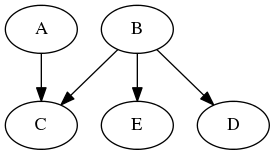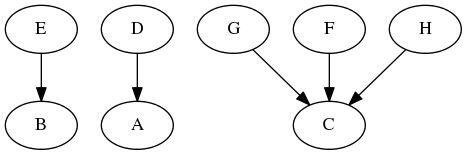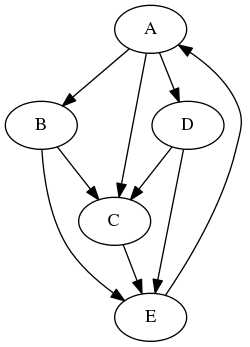# Exercise 2.3¶

The exercises in this IPython notebook are based on the exercises of Chapter 14 of Easly and Kleinberg (2010).

Let's recreate the graph of Figure 14.15 from the book, and display its image:

In :
import networkx as nx
import IPython

G1 = nx.DiGraph()

viz = nx.to_agraph(G1)
viz.layout(prog='dot')
viz.draw('figure14-15.png')

IPython.display.Image('figure14-15.png')

Out:## Exercise 2.3.1, the Authority Update Rule¶

Implement the function authority_update(Graph, node_values) that returns the values that you get if you run the Authority Update Rule on the network Graph, see Page 356 of Easly & Kleinberg. The values should be returned in a Python dictionary with nodes as key and values the sum of the hub scores of all pages that point to the node.

Tip: The suggested solution below contains a third parameter normalized that has a default value (False). This third parameter is not mandatory when calling the function. You can ignore the third parameter for now. We will use it in Exercise 2.3.4.

In :
# Produces a dictionary with nodes as key and values 1
# Can also be done with list comprehension as: start_values = { node : 1 for node in G1 }
start_values_G1 = {}
for node in G1:
start_values_G1[node] = 1

# This function should implement the authority update rule.
def authority_update (Graph, node_values, normalized=False):
authority_values = {}
#
#
return authority_values

authorities = authority_update(G1, start_values_G1)

authorities  # show the result

Out:
{}

## Exercise 2.3.2, the Hub Update Rule¶

Implement the function hub_update(Graph, node_values) that returns the values that you get if you run the Hub Update Rule on the network Graph.

In :
# This function should implement the authority update rule.
def hub_update (Graph, node_values, normalized=False):
hub_values = {}
#
#
return hub_values

hubs = hub_update(G1, authorities)

hubs # show the result

Out:
{}

## Exercise 2.3.3, Hub Authority updates¶

Implement the function hub_authority_updates(Graph, node_values, k, normalized=False) that performs a sequence of k hub-authority updates, as explained on Page 357 of Easly & Kleinberg. The function should return the hub values of each node, and also the authority values of each node.

Tip: Note that Kleinberg maybe should have called the algorithm: "Authority Hub Updates"...

In :
def hub_authority_updates(Graph, node_values, k, normalized=False):
authority_values = {}
hub_values = {}
#
#
return hub_values, authority_values


Out:
({}, {})

## Exercise 2.3.4, Normalization¶

Go back to your solutions of Exercises 2.3.1, 2.3.2, and 2.3.3, and implement normalization by adding the following to your implementation:

if (normalized):
#
#



Tip: Make sure that hub_authority_updates() passed the value of normalized on to the update functions.

## Another graph¶

Let's have a look at the graph of Figure 14.16 of the book.

In :
G2 = nx.DiGraph()

viz = nx.to_agraph(G2)
viz.layout(prog='dot')
viz.draw('figure14-16.png')

IPython.display.Image('figure14-16.png')

Out:## Exercise 2.3.5, again¶

Run hub_authority_updates() on graph G2, for k=2 with and without normalization.

Tip: See Exercise 2a on Page 378 of Easly & Kleinberg.

In :
start_values_G2 = { node : 1 for node in G2 }

#
#


## Exercise 2.3.6, add an edge¶

What happens to the scores when node 'E', which links to 'B', decides to link to 'C' as well? Which of the nodes 'A' or 'B' now has the higher authority score? Give a brief explanation in which you provide some inuition for why the difference in authority scores between 'A' and 'B' turned out the way it did.

Tip: See Exercise 2b and 2c on Page 379 of Easly & Kleinberg.

In :
G3 = G2.copy()

#
#


## Exercise 2.3.7, bonus¶

Use your implementation of the Hub-Authority Algorithm to check your solutions for Exercise 3 on Pages 379 and 380, and Exercise 6 on Page 382 of Easly & Kleinberg.

## Exercise 2.3.8, with NetworkX¶

Search the NetworkX Reference Documentation for the hub-authority algorithm. Show the values for hubs and authorities that you get by running the k-step hub-authority algorithm provided by NetworkX, when we choose the number of steps k to be 2. Run the NetworkX algorithm on G1, G2, and G3, with and without normalization.

Do both implementations give the same results? Which implementation is closer to the implementation presented by Easly & Kleinberg?

In :
#
#


## PageRank¶

Consider the graph from Figure 14.19 from Easly & Kleinberg:

In :
G4 = nx.DiGraph()

viz = nx.to_agraph(G4)
viz.layout(prog='dot')
viz.draw('figure14-19.png')

IPython.display.Image('figure14-19.png')

Out:## Exercise 2.3.9, NetworkX PageRank¶

From the NetworkX Documentation (See: Link Analysis), use the PageRank algorithm to check if the assignment of the numbers to the nodes in Figure 14.19 of Easly & Kleinberg on Page 381 form an equilibrium set of the PageRank values for this network of web pages. Explain your answer.

In :
#
#


## Exercise 2.3.10, Bonus 1¶

Also check your answers for the graphs in Figures 14.20 and 14.21 of Easly & Kleinberg.

In :
#
#


## Exercise 2.3.11, Bonus 2: Implement your own PageRank¶

Implement your own version of PageRank.

Tip: Take inspiration from the function authority_update() above.

In :
#

This is a very big graph that might be too big for your system to handle. There are two versions of the graph on Blackboard: utwente201509.dot and utwente201509small.dot: The latter does not contain the URLs, making it somewhat easier to handle for NetworkX.